# Euclidean Distance Matrix

Step by step explanation on how EDM is represented in linear algebra and how to code it as a function in Python in just one line.Pitagora : Euclide = Triangle : Geometry (drawing by : Andrea Grianti)

Hi everybody, in this post I want to explain my experience in figuring out how, a rather intuitive concept like that of the Euclidean Distance Matrix (EDM), could become a challenge if you decide to improve your (in my case Python) programming skills crossing the chasm from classical “for…loops” type of code toward the beauty of a single line of code using linear algebra concepts.

Why ? Because if you can solve a problem in a more efficient way with one line of code but you don’t understand how to do it because you do not have linear algebra skills, well it’s time to learn and try.

The result is amazing and elegant but best of all opens up your mind in thinking with vectors and matrices which is important if you want to move later to other data science topics.

Hi everybody my name is Andrea Grianti, I spent my professionial life in IT and Data Warehousing but I became later more and more passionate with data science and analytics topics.

## What is Euclidean Distance Matrix (EDM)

An easy “distance table” to imagine is that in a map with cities in both rows and columns with miles in the crossing cells representing a distance concept. In that case an EDM is often seen as a squared table (matrix) where the diagonal is zero (i.e. distance between same city is zero) and the rest of the table is symmetrical (distance from a to b is the same as distance from b to a).

Even the ‘distance’ concept is also not trivial as there are many different ways to define a ‘distance’. Here I consider distance the euclidean one which is usually represented by something like this: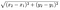formula to calculate distance in two dimensions from point A to point B. elements of the formula are the projections of the vectora A and B over the two dimensions axis.

But even this simple formula could easily become a lot more complex if you think data tables with thousands of rows on one side and hundreds of “features” (n-dimensional space). It’s here that becomes evident the gap between thinking “code” and thinking “algebra”.

Rows and Columns mean for most of us something like this in code:

But this is exactly what we want to avoid and we want to find a smarter way to operate with tables minimizing the code to write.

# The starting point

To keep it simple suppose you have a matrix A (4,2) and matrix B (3,2). A is made of 4 rows (think about players) with 2 features, B is 3 rows and same 2 features.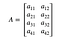Matrix A(4,2), each row can be observations of two distinct features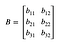Matrix B(3,2). A and B share the same dimensional space. In this case 2. So the dimensions of A and B are the same.

We want to calculate the euclidean distance matrix between the 4 rows of Matrix A from the 3 rows of Matrix B and obtain a 4x3 matrix D where each cell represents the distance between a vector in matrix A and a vector in matrix B. I call for example a1 the vector with elements (a11,a12); b1 is the vector (b11,b12)etc. etc.: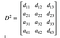From now on we work on squared values and we leave the ‘square root’ as a last passage of our procedure

It’s important to note that nothing change if you instead have a unique matrix (let’s say A) and you want to calculate the distances between each point of that matrix. It’s the same problem with A=B.

In our case we can define each cell element of the D matrix as: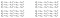each cell is nothing difficult as it’s the classical squared distance between points in a n-dimensional space

if we analyze in our analysis just the first element (d11) we find out the following :

1. In equation (1) we have just developed the polynomial and we discover interesting things I colored in red, blue and black to better highlight. In red: that sum is just what the mathematicians call the norm-2 (here is squared) of the vector 1 (let’s call it a1) of our matrix A.
2. If you don’t know what the norm-2 is just look at equations number 2 and 3. It’s written in the books most of the times in that way and it’s simply the sum of the squares of the elements of a vector. In this case our first vector a1. Don’t forget that the norm formula operates also a square root function on the sum of squares. But in order to proceed we leave it out for the moment and the reason is : we can better see the diagonal property of the dot product between two matrices.

3. In blue: we are still analyzing equation1 and this is similar to the red part but for the vector 1 (call it b1) of our matrix B.

4. In black: see equation 4. We recognize here the classical dot product of two vectors (you remember the elementary [rows] by [columns] operation) so we can write in that way because it’s the dot product between row vector a1 and column (it was a row but we transposed it) vector b1. T is to mean that you must transpose the vector b1 in order to make it possible multiply it with the vector a1.

If we rewrite our Full Euclidean Distance Matrix with all the cells “exploded” and written as we did for the first element we have the following: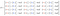Take a moment to see the patterns and try to focus on how to generalize them.

You see the patterns in the matrix ? First of all we can check that D is actually the sum of three parts as follow:

1. the red columns repeat themselves vertically =>therefore we can isolate that apart as (for now) a vertical vector made of all the norms of each horizontal row of the A matrix.
2. the blue rows are conceptually similar to the red columns even if in this case you will notice that they repeat themselves horizontally top-down
3. the black is also intersting because it’s the dot product of our full original matrices A and B (transposed) multiplied by the scalar -2. Matrix A was (4 by 2) and B was (3 by 2) so B transposed is (2 by 3) and -2 times A dot product B is (4 by 3).

So we can say that matrix D is the sum of three parts but we have to tell more about how to calculate the norm-2 of the first two components: the red and blue parts. We have said that the red part is the sum of the squares of the row vectors of the matrix A, while the blue part is the sum of the squares of the row vectors of matrix B (before transposing).

In matrix operations terms you expect to obtain 2 columns. One column of values for A and one for B. You can check that the result we are looking for comes from the main diagonal values of dot product of matrix A by matrix A transposed.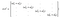Highlights of the elements of the diagonal . The same applies to the B matrix.

so we can say that: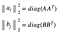if you think in python you can obtain the same result by squaring and summing A. This is the typical case when you could decide to take a shortcut and leave the tracks of algebra for something easier.

One more thing: in order to make it possible summing the three elements we must be sure they can be summed. This means that we have a little work to do on the red and blue parts to make them compatibles with the result of the dot product which is 4 by 3.

To make the two norms columns like rectangular matrices so that they can be summed all together we know that the produt of a column (in this case 4 by 1) and a row of an identity matrix (here 1 by 3) “replicates” the original column creating a matrix of 3 identical columns .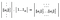the concept of broadcasting in matrix literature is achieved through the multiplication of a vector with a right sized identity vector and the result is a â€œrepeatâ€ of the column m times. The same concept applies the other way round to a row vector multiplied by a column identity vector. This is another situation where following algebra with coding becomes inefficient. So think with an end in mind and follow your intuition. In Python for example read about the concept of ‘broadcasting’ and you can forget to code identity matrices.

The similar thing happens with the column vector of B which is 3 rows by 1 column. In this case we first transpose the column and multiply it for a identity vector of 4 rows by 1.

This looks complex but it’s not because when we think in Python we can leverage the broadcasting feature and so we can avoid this approach alltogether, but if you want to understand algebra here we are.

Well in the end of the end we have all the parts which are compatible for being summed and found out the solution can be generalized like this which is often reported in many web sites :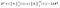The general formula for EDM. Square root operation can be done as a very last passage.

How to translate that in Python:

The first 2 parts of the D² equation for the general formula are matrices where the norms have been “repeated” in order to make it possible summing them with -2AB^T. You can calc those matices in several ways. The point is that if you code it exactly following the algebra formulas => with identity matrix and such, the code is not efficient because you have probably to transpose, reshape, calculate the diagonals with functions like np.diag, np.reshape(…) etc.,. If you want to do it as an excercise, it works.

Instead the following code leverages Python features to do the same and can be squeezed in a single line of code (!). It accepts as input 2 matrices and returns a matrix with the distances. No for…loops or similar things.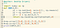if you like you can actually put all the code in the return statement. depending on the needs I decided to round the results with 2 decimals, but you can take that out if you don’t like it.

That’s all. I hope you liked it and your comments are very welcome.

## The Startup

Get smarter at building your thing. Join The Startup’s +724K followers.

Written by

## Andrea Grianti

IT Senior Manager and Consultant. Data Warehouse and Business Intelligence expertise in design and build. Freelance.

## The Startup

Get smarter at building your thing. Follow to join The Startup’s +8 million monthly readers & +724K followers.

Written by

## Andrea Grianti

IT Senior Manager and Consultant. Data Warehouse and Business Intelligence expertise in design and build. Freelance.## The Startup

Get smarter at building your thing. Follow to join The Startup’s +8 million monthly readers & +724K followers.

## More From Medium

Medium is an open platform where 170 million readers come to find insightful and dynamic thinking. Here, expert and undiscovered voices alike dive into the heart of any topic and bring new ideas to the surface. Learn more

Follow the writers, publications, and topics that matter to you, and you’ll see them on your homepage and in your inbox. Explore

If you have a story to tell, knowledge to share, or a perspective to offer — welcome home. It’s easy and free to post your thinking on any topic. Write on Medium